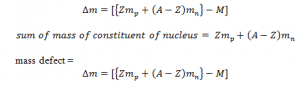# Mass Defect

Nucleus consists of proton and neutron the sum of the mass of proton and neutron gives the mass of the nucleus ( exact mass) .
However, the mass of the nucleus is always less than the sum of the mass of constituent of nucleus. The difference in ass is known as mass defect. Let z be the no. of proton and Mp be the mass of the proton , A be total no. of constituent and Mn be the mass of neutron and ‘M’ be the mass of nucleus:This difference in mass appears. It is because this mass is converted to energy. According to energy mass relation.
E=mc2. This energy is known as binding energy. (E=mc2)